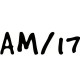Class 10 Maths Notes

# CBSE Class 10 Maths Chapter 7 Coordinate GeometryWritten by
Coordinate GeometryCoordinate Geometry

### Coordinate Geometry

To locate the position of a point on a plane, we require a pair of coordinate axes. The distance of a point from the y-axis is called its x-coordinate, or abscissa. The distance of a point from the x-axis is called its y-coordinate, or ordinate. The coordinates of a point on the x-axis are of the form (x, 0), and of a point on the y-axis are of the form (0, y).Coordinate geometry is used in creating maps. It is also used in creating digital images. It has huge application in the field of architecture, physics, engineering, navigation, seismology and art.

### Distance FormulaThe distance of a point P(xy) from the origin is √x2 + y2 .
Numerical: Find the distance between the following pairs of points (2, 3), (4, 1)
Solution: here x1 =2, y1 = 3 , x2 = 4 & y2 =1### Section FormulaSo, the coordinates of the point P(x, y) which divides the line segment joining the points A(x1, y1) and B(x2, y2), internally, in the ratio m1 : m2 are { (m1x2 + m2x1)/(m1 + m2 ) ,  (m1y2 + m2y1)/(m1 + m2 ) } .This is known as the section formula.
Refer ExamFear video lessons for Proof
The mid-point of the line segment joining the points P(x1y1) and Q(x2y2) is [(x1+x2)/2 ,(y1+y2)/2]
Numerical: Find the coordinates of the point which divides the line segment joining the points (4, – 3) and (8, 5) in the ratio 3 : 1 internally
Solution: Using section formula P(x, y) = { (m1x2 + m2x1)/(m1 + m2 ) ,  (m1y2 + m2y1)/(m1 + m2 ) }
We get P(x, y) = { (3*8 + 1*4 )/(3+1)   ,   (3* 5  + 1 *-3)/(3+1)   }   = (7,3)

### Area of a Triangle

Area of Δ ABC, with A(x1,y1) , B(x2, y2)  & C(x3,y3) is the given by      ½ [ x1(y2-y3)  + x2 (y3-y1 ) + x3 (y1-y2)]Numerical: Find the area of a triangle whose vertices are (1, –1), (– 4, 6) and (–3, –5).
Solution:
Area of Δ ABC, with A(x1,y1) , B(x2, y2)  & C(x3,y3) is the given by      ½ [ x1(y2-y3)  + x2 (y3-y1 ) + x3 (y1-y2)]
Using this, Area of Triangle is  ½ [1*(6+5) + (-4)* (-5 + 1) + (-3)* (-1-6) ]

Or Area = ½ [11+ 16 + 21]    = 24 square units.

### MATHS Revision Notes

Chapter:01  Real Numbers System
Chapter:02  Polynomials
Chapter:03  Pair of Linear Equations in Two Variables
Chapter:05  Arithemetic Progressions
Chapter:06  Triangles
Chapter:07  Coordinate Geometry
Chapter:08  Introduction to Trignometry
Chapter:09  Some Application Of Trignometry
Chapter:10  Circles
Chapter:11  Constructions
Chapter:12  Area Related to Cirles
Chapter:13  Surface Area Volume
Chapter:14  Stastistics
Chapter:15  Probability

Science Revision Notes

English Revision Notes

Economics Revision Notes